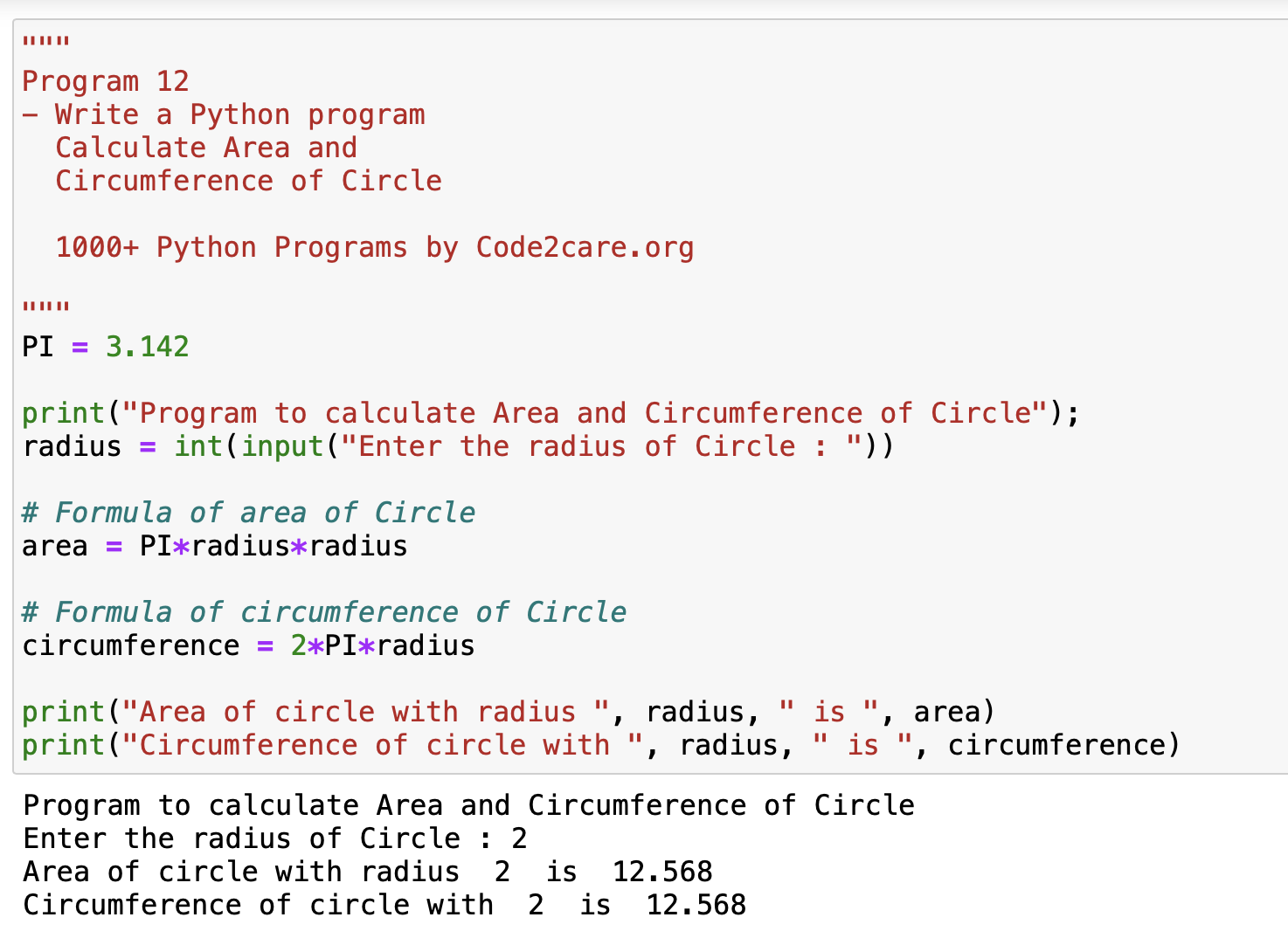# Program 12: Calculate Area and Circumference of Circle - 1000+ Python Programs

In this program number 12 in our series, let's calculate the area of a circle, as well as its circumference.

Formula:
``````Area of Circle: π x r2

Circumference of Circle: 2 x π x r``````

As the value of PI is a constant 3.14 all that we need to get from the user as input is the radius of the circle.

## Python Code

``````"""
Program 12
- Write a Python program
Calculate Area and
Circumference of Circle

1000+ Python Programs by Code2care.org

"""

PI = 3.142

print("Program to calculate Area and Circumference of Circle");

# Formula of the area of Circle

# Formula of the circumference of Circle

print("The Area of a circle with radius ", radius, " is ", area)
print("Circumference of a circle with ", radius, " is ", circumference)``````
Output Example:

Program to calculate the Area and Circumference of Circle
Enter the radius of Circle: 10
The area of a circle with a radius of 10 is 314.2
Circumference of a circle with 10 is 62.839999999999996

## Python build-in functions used in this program

• print() - an inbuild function in Python 3 - read more, we can also use of the f-strings along with print function to get the desired result.
• input(prompt) - an inbuild function again, with the prompt string that is printed out to the standard output without a trailing newline - read more-

Have Questions? Post them here!